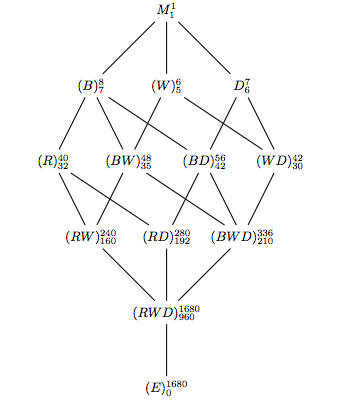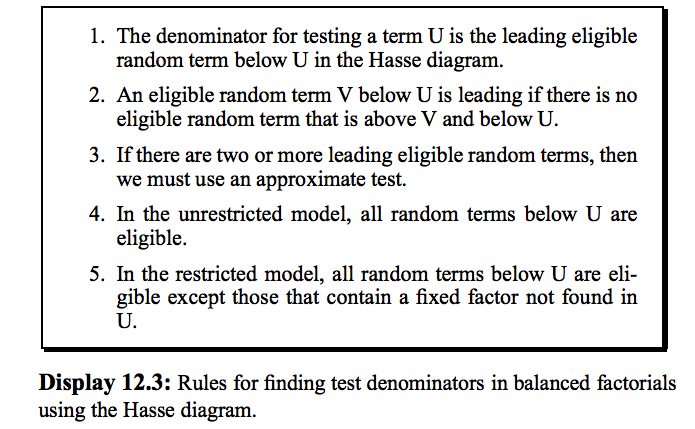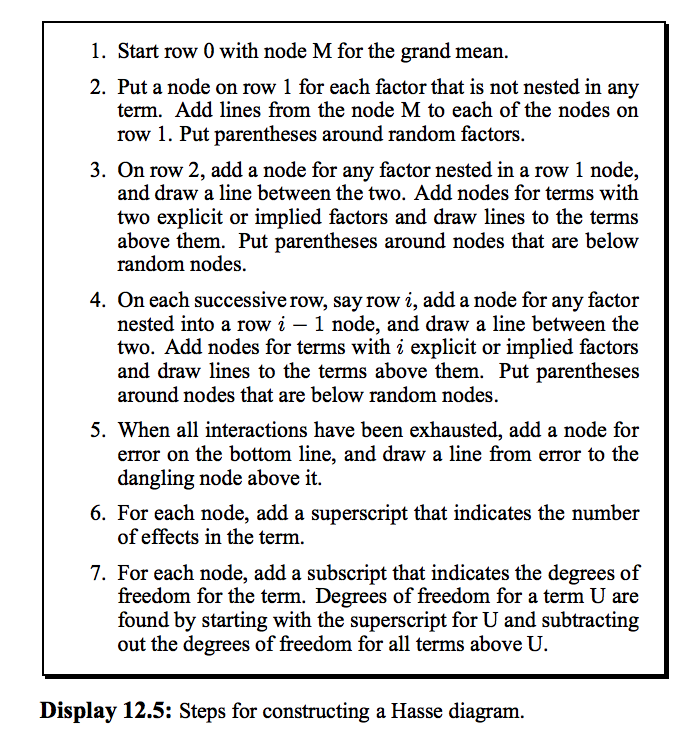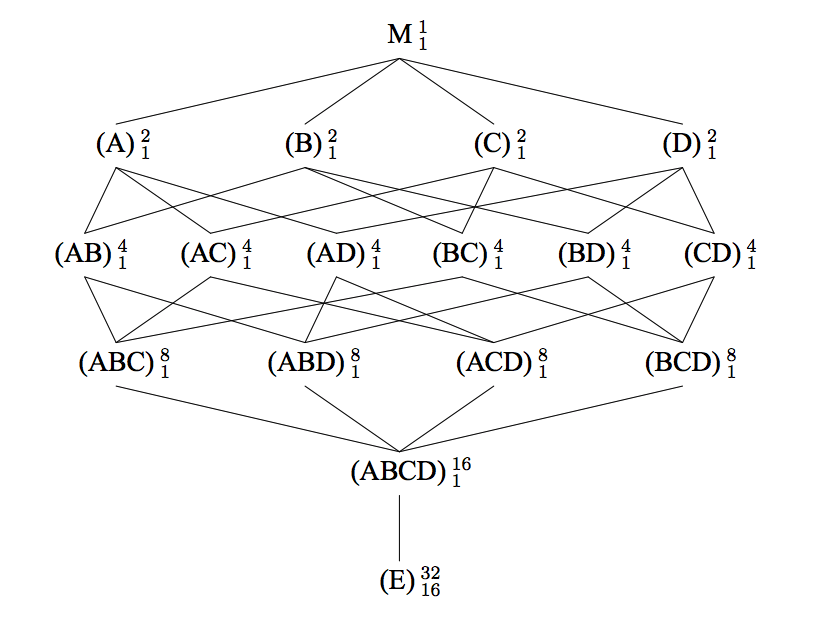Design and Analysis of Experiments
Post
Cancel

# Design and Analysis of Experiments

This is my notes of the course STAT 8052 and of the book: A first Course in Design and Analysis of Experiments.

## Book Notes

### Chapter 12

1. How to tell random or fixed? Do the experiment again, will the levels of the treatment change or not? Random effect is randomly sampled from a population.
2. How to tell nested or crossed? Consider A and B. If the 1st level of A in B1 has nothing to do with the 1st level of A in B2, then nested. If A contains all levels of B and B contains all levels of A, then A and B are crossed. So if C is nested in B and that C & D are crossed, then B & D are also crossed.
3. Note that (R) and (WD)are not added in the 4th layer, but in the 5th layer. I think the reason is that when we add (RW) and (RD), we will also get (RWD). If we do that in the 4th layer, then the product and the seperate elements will be in the same layer. Notice: the numbers of RWD should be reversed. Similar case also happens in Problem 12.3 in the book.4.5.6.#### 12.6 Hasse Diagrams and Expected Mean SquaresThe approximate test for term A is: $\frac{A + ABC + ABD + ACD}{AB + AC + AD + ABCD}$.

## Coding Notes

1. After reading the data, do remember use as.factor().
2. When compling Rmarkdown, even a blank line will make an error.

## Generalized Linear Fixed Models

1. When using glmer() to fit a GLMM model, check the following things:
• Is rescaling needed for numerical variable? Use either scale() or manually rescale for the purpose of interpretation.
• Is the iteration times large enough and the tolerance for grad too small? Try use “control” argument. control = glmerControl(check.conv.grad = .makeCC(action = "message", tol = 0.01), optCtrl = list(maxfun = 10000)).
• Check this lme4 convergence warnings: troubleshooting for more detail.
• Check the reference level. Changing the reference level would help the convergence.
2. When using geeglm():
• It only works when the response is numerical. So don’t set the response to be a factor, even for binary response. And it’s useless to use as.numeric() to reverse the factor to the numerical in this case. Recreating the data would work. Check this post for more detail.
3. Check the reference level.
• The result might be different when we use different reference levels. For example, in Faraway Chapter 13 Problem 4, treating brood 1 and brood 3 as reference will give totally different results.
 nitro2 <- nitro
nitro2 <- within(nitro2, brood <- relevel(brood, ref = 3))